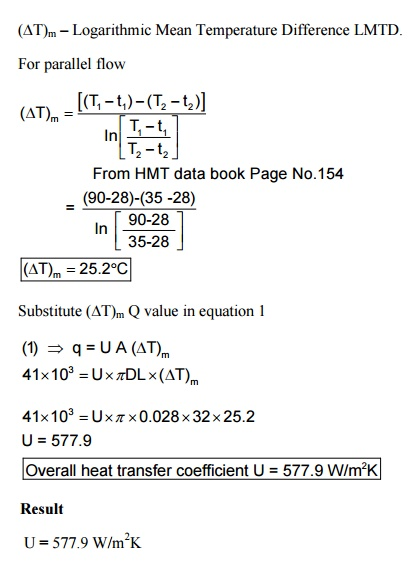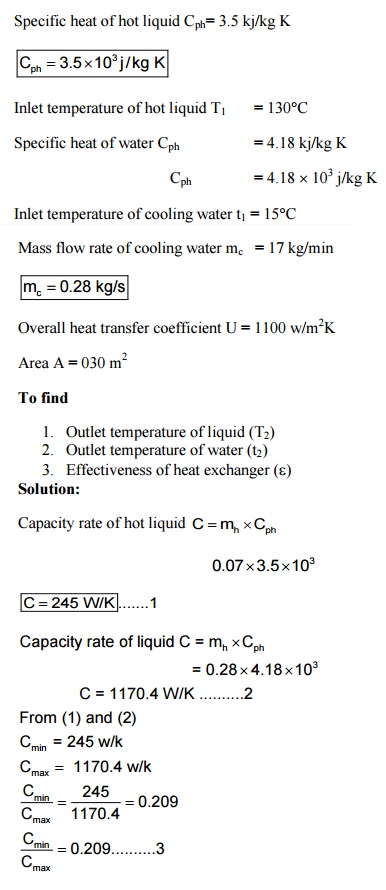Home | | Heat and Mass Transfer | Solved Problems - Heat and Mass Transfer - Radiation

# Solved Problems - Heat and Mass Transfer - Radiation

Mechanical - Heat and Mass Transfer - Radiation

1. In a counter flow double pipe heat exchanger, oil is cooled from 85°C to 55°C by water entering at 25°C. The mass flow rate of oil is 9,800 kg/h and specific heat of oil is 2000 j/kg K. the mass flow rate of water is 8,000 kg/h and specific heat of water is 4180 j/kg K. Determine the heat exchanger area and heat transfer rate for an overall heat transfer coefficient of 280 W/m2 K.

Given:

Hot fluid –oil        Cold fluid –water

T1,T2                   t1, t2

Entry temperature of oil T1 = 85°C

Exit temperature of oil   T2 = 55°C

Entry temperature of water t1 = 25°C

Mass flow rate of oil (Hot fluid) mh = 9,800 kg/hSpecific heat of water Cpc –4180 j/kg K

Overall heat transfer coefficient U = 280 W/m2K

To find

1.     Heat exchanger area (A)

2.     Heat transfer rate (Q)

Solution

We know that

Heat lost by oil Hot fluid = Heat gained by water cold fluid

Qh = Qc(1) Þ Q = UA (Delta T)m

Þ   162 ´103   =280  A´ 35´.8

Þ    A = 16.16 m2

2.An oil cooler of the form of tubular heat exchanger cools oil from a temperature of 90°C to 35°C by a large pool of stagnant water assumed constant temperature of 28°C. The tube length is 32 m and diameter is 28 mm. The specific heat and specific gravity of the oil are 2.45 kj/kg K and 0.8 respectively. The velocity of the oil is 62 cm/s. Calculate the overall heat transfer coefficient.A parallel flow heat exchanger is used to cool. 4.2 kg/min of hot liquid of specificheat 3.5 kj/kgK at 130°C. A cooling water of specific heat 4.18 kj/kg K is used for cooling purpose at a temperature of 15°C. The mass flow rate of cooling water is 17 kg/min calculate the following.

1. Outlet temperature of liquid

2. Outlet temperature of water

3. Effectiveness of heat exchanger Take care,

Overall heat transfer coefficient is 1100 W/m2 K.

Heat exchanger area is 0.30 m2

Given:

Mass flow rate of hot liquid mh= 4.2 kg/min

M h = 0.07 kg/s

Specific heat of hot liquid Cph= 3.5 kj/kg K5. In a cross heat exchangers both fluids unmixed hot fluid with a specific heat of 2300 J/kg K enters at 380°C and leaves at 300°C cold fluids enters at 25°C and leaves at 210°C. Calculate the required surface of heat exchanger. Take overall heat transfer coefficient is

750 W/m2 K. Mass flow rate of hot fluid is 1 kg/s.

Given:

Specific heat of hot fluid Cph  = 23000 J/kg KStudy Material, Lecturing Notes, Assignment, Reference, Wiki description explanation, brief detail
Mechanical : Heat and Mass Transfer : Phase Change Heat Transfer and Heat Exchangers : Solved Problems - Heat and Mass Transfer - Radiation |